Hostname: page-component-546b4f848f-hhr79 Total loading time: 0 Render date: 2023-06-02T09:51:33.707Z Has data issue: false Feature Flags: { "useRatesEcommerce": true } hasContentIssue false

# Wake structure of laminar flow past a sphere under the influence of a transverse magnetic field

Published online by Cambridge University Press:  20 June 2019

## Abstract

The wake structure of an incompressible, conducting, viscous fluid past an electrically insulating sphere affected by a transverse magnetic field is investigated numerically over flow regimes including steady and unsteady laminar flows at Reynolds numbers up to 300. For a steady axisymmetric flow affected by a transverse magnetic field, the wake structure is deemed to be a double plane symmetric state. For a periodic flow, unsteady vortex shedding is first suppressed and transitions to a steady plane symmetric state and then to a double plane symmetric pattern. Wake structures in the range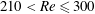$210<Re\leqslant 300$ without a magnetic field have a symmetry plane. An angle$\unicode[STIX]{x1D703}$ exists between the orientation of this symmetry plane and the imposed transverse magnetic field. For a given transverse magnetic field, the final wake structure is found to be independent of the initial flow configuration with a different angle$\unicode[STIX]{x1D703}$. However, the orientation of the symmetry plane tends to be perpendicular to the magnetic field, which implies that the transverse magnetic field can control the orientation of the wake structure of a free-moving sphere and change the direction of its horizontal motion by a field–wake–trajectory control mechanism. An interesting ‘reversion phenomenon’ is found, where the wake structure of the sphere at a higher Reynolds number and a certain magnetic interaction parameter (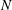$N$) corresponds to a lower Reynolds number with a lower$N$ value. Furthermore, the drag coefficient is proportional to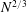$N^{2/3}$ for weak magnetic fields or to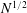$N^{1/2}$ for strong magnetic fields, where the threshold value between these two regimes is approximately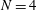$N=4$.

Type
JFM Papers
Information
Journal of Fluid Mechanics , 25 August 2019 , pp. 151 - 173

## Access options

Get access to the full version of this content by using one of the access options below. (Log in options will check for institutional or personal access. Content may require purchase if you do not have access.)

## References

Branover, H., Eidelman, A. & Nagorny, M. 1995 Use of turbulence modification for heat transfer enhancement in liquid metal blankets. Fusion Engng Des. 27, 719724.10.1016/0920-3796(94)00272-9CrossRefGoogle Scholar
Chester, W. 1957 The effect of a magnetic field on Stokes flow in a conducting fluid. J. Fluid Mech. 3 (3), 304308.10.1017/S0022112057000671CrossRefGoogle Scholar
Davidson, P. A. 2001 An Introduction to Magnetohydrodynamics. Cambridge University Press.10.1017/CBO9780511626333CrossRefGoogle Scholar
Delacroix, J. & Davoust, L. 2018 Drag upon a sphere suspended in a low magnetic-Reynolds number MHD channel flow. Phys. Rev. Fluids 3, 123701.10.1103/PhysRevFluids.3.123701CrossRefGoogle Scholar
El-Kaddah, N., Patel, A. D. & Natarajan, T. T. 1995 The electromagnetic filtration of molten aluminum using an induced-current separator. JOM J. Miner. Met. Mater. Soc. 47 (5), 4649.10.1007/BF03221176CrossRefGoogle Scholar
Ern, P., Risso, F., Fabre, D. & Magnaudet, J. 2012 Wake-induced oscillatory paths of bodies freely rising or falling in fluids. Annu. Rev. Fluid Mech. 44, 97121.10.1146/annurev-fluid-120710-101250CrossRefGoogle Scholar
Fabre, D., Auguste, F. & Magnaudet, J. 2008 Bifurcations and symmetry breaking in the wake of axisymmetric bodies. Phys. Fluids 20 (5), 051702.10.1063/1.2909609CrossRefGoogle Scholar
Frank, M., Barleon, L. & Müller, U. 2001 Visual analysis of two-dimensional magnetohydrodynamics. Phys. Fluids 13 (8), 22872295.10.1063/1.1383785CrossRefGoogle Scholar
Ghidersa, Brăduţ & Dušek, J. 2000 Breaking of axisymmetry and onset of unsteadiness in the wake of a sphere. J. Fluid Mech. 423, 3369.10.1017/S0022112000001701CrossRefGoogle Scholar
Goldsworthy, F. A. 1961 Magnetohydrodynamic flows of a perfectly conducting, viscous fluid. J. Fluid Mech. 11 (4), 519528.10.1017/S0022112061000706CrossRefGoogle Scholar
Horowitz, M. & Williamson, C. H. K. 2010 The effect of Reynolds number on the dynamics and wakes of freely rising and falling spheres. J. Fluid Mech. 651, 251294.10.1017/S0022112009993934CrossRefGoogle Scholar
Jenny, M., Dušek, J. & Bouchet, G. 2004 Instabilities and transition of a sphere falling or ascending freely in a Newtonian fluid. J. Fluid Mech. 508, 201239.10.1017/S0022112004009164CrossRefGoogle Scholar
Johnson, T. A. & Patel, V. C. 1999 Flow past a sphere up to a Reynolds number of 300. J. Fluid Mech. 378, 1970.10.1017/S0022112098003206CrossRefGoogle Scholar
Kalis, K., Slyusarev, N., Tsinober, A. & Shtern, A. 1966 Resistance of bluff bodies at high Stewart numbers. Magnetohydrodynamics 2 (4), 152153.Google Scholar
Kanaris, N., Albets, X., Grigoriadis, D. & Kassinos, S. 2013 Three-dimensional numerical simulations of magnetohydrodynamic flow around a confined circular cylinder under low, moderate, and strong magnetic fields. Phys. Fluids 25 (7), 074102.10.1063/1.4811398CrossRefGoogle Scholar
Lahjomri, J., Capéran, P. & Alemany, A. 1993 The cylinder wake in a magnetic field aligned with the velocity. J. Fluid Mech. 253, 421448.10.1017/S0022112093001855CrossRefGoogle Scholar
Lighthill, M. J. 1963 Boundary layer theory. In Laminar Boundary Layers (ed. Rosenhead, L.), pp. 46103. Oxford University Press.Google Scholar
Maxworthy, T. 1962 Measurements of drag and wake structure in magneto-fluid dynamic flow about a sphere. In Heat Transfer and Fluid Mechanics Institute Annual Meeting, pp. 197205. Stanford University Press.Google Scholar
Mittal, R. 1999 Planar symmetry in the unsteady wake of a sphere. AIAA J. 37 (3), 388390.10.2514/2.722CrossRefGoogle Scholar
Moreau, R. J. 2013 Magnetohydrodynamics. Springer.Google Scholar
Mück, B., Günther, C., Müller, U. & Bühler, L. 2000 Three-dimensional MHD flows in rectangular ducts with internal obstacles. J. Fluid Mech. 418, 265295.10.1017/S0022112000001300CrossRefGoogle Scholar
Müller, U. & Bühler, L. 2013 Magnetofluiddynamics in Channels and Containers. Springer.Google Scholar
Mutschke, G., Gerbeth, G., Shatrov, V. & Tomboulides, A. 2001 The scenario of three-dimensional instabilities of the cylinder wake in an external magnetic field: a linear stability analysis. Phys. Fluids 13 (3), 723734.10.1063/1.1344895CrossRefGoogle Scholar
Natarajan, R. & Acrivos, A. 1993 The instability of the steady flow past spheres and disks. J. Fluid Mech. 254, 323344.10.1017/S0022112093002150CrossRefGoogle Scholar
Ni, M.-J., Munipalli, R., Huang, P., Morley, N. B. & Abdou, M. A. 2007 A current density conservative scheme for incompressible MHD flows at a low magnetic Reynolds number. Part II: On an arbitrary collocated mesh. J. Comput. Phys. 227 (1), 205228.10.1016/j.jcp.2007.07.023CrossRefGoogle Scholar
Ormières, D. & Provansal, M. 1999 Transition to turbulence in the wake of a sphere. Phys. Rev. Lett. 83 (1), 8083.10.1103/PhysRevLett.83.80CrossRefGoogle Scholar
Pan, J.-H., Zhang, N.-M. & Ni, M.-J. 2018 The wake structure and transition process of a flow past a sphere affected by a streamwise magnetic field. J. Fluid Mech. 842, 248272.10.1017/jfm.2018.133CrossRefGoogle Scholar
Pan, J.-H., Zhang, N.-M. & Ni, M.-J. 2019 Instability and transition of a vertical ascension or fall of a free sphere affected by a vertical magnetic field. J. Fluid Mech. 859, 3348.10.1017/jfm.2018.803CrossRefGoogle Scholar
Pier, B. 2008 Local and global instabilities in the wake of a sphere. J. Fluid Mech. 603, 3961.10.1017/S0022112008000736CrossRefGoogle Scholar
Sarris, I. E., Zikos, G. K., Grecos, A. P. & Vlachos, N. S. 2006 On the limits of validity of the low magnetic Reynolds number approximation in MHD natural-convection heat transfer. Numer. Heat Transfer 50 (2), 157180.10.1080/10407790500459403CrossRefGoogle Scholar
Sekhar, T. V. S., Sivakumar, R. & Kumar, T. R. 2005 Magnetohydrodynamic flow around a sphere. Fluid Dyn. Res. 37 (5), 357373.10.1016/j.fluiddyn.2005.08.003CrossRefGoogle Scholar
Shatrov, V., Mutschke, G. & Gerbeth, G. 1997 Numerical simulation of the two-dimensional flow around a circular cylinder. Magnetohydrodynamics 33 (1), 210.Google Scholar
Tomboulides, A. G. & Orszag, S. A. 2000 Numerical investigation of transitional and weak turbulent flow past a sphere. J. Fluid Mech. 416, 4573.10.1017/S0022112000008880CrossRefGoogle Scholar
Yonas, G. 1967 Measurements of drag in a conducting fluid with an aligned field and large interaction parameter. J. Fluid Mech. 30 (4), 813821.10.1017/S002211206700179XCrossRefGoogle Scholar
Zheng, T. X., Zhong, Y. B., Lei, Z. S., Ren, W. L., Ren, Z. M., Debray, F., Beaugnon, E. & Fautrelle, Y. 2015 Effects of high static magnetic field on distribution of solid particles in BiZn immiscible alloys with metastable miscibility gap. J. Alloys Compds 623, 3641.10.1016/j.jallcom.2014.10.095CrossRefGoogle Scholar